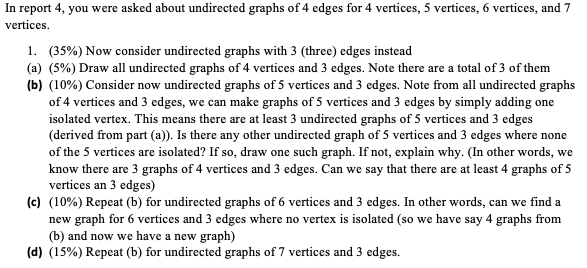# (Solved) : Report 4 Asked Undirected Graphs 4 Edges 4 Vertices 5 Vertices 6 Vertices 7 Vertices 1 35 Q42773508 . . .In report 4, you were asked about undirected graphs of 4 edges for 4 vertices, 5 vertices, 6 vertices, and 7 vertices. 1. (35%) Now consider undirected graphs with 3 (three) edges instead (a) (5%) Draw all undirected graphs of 4 vertices and 3 edges. Note there are a total of 3 of them (b) (10%) Consider now undirected graphs of 5 vertices and 3 edges. Note from all undirected graphs of 4 vertices and 3 edges, we can make graphs of 5 vertices and 3 edges by simply adding one isolated vertex. This means there are at least 3 undirected graphs of 5 vertices and 3 edges (derived from part (a)). Is there any other undirected graph of 5 vertices and 3 edges where none of the 5 vertices are isolated? If so, draw one such graph. If not, explain why. (In other words, we know there are 3 graphs of 4 vertices and 3 edges. Can we say that there are at least 4 graphs of 5 vertices an 3 edges) (c) (10%) Repeat (b) for undirected graphs of 6 vertices and 3 edges. In other words, can we find a new graph for 6 vertices and 3 edges where no vertex is isolated (so we have say 4 graphs from (b) and now we have a new graph) (d) (15%) Repeat (b) for undirected graphs of 7 vertices and 3 edges. Show transcribed image text In report 4, you were asked about undirected graphs of 4 edges for 4 vertices, 5 vertices, 6 vertices, and 7 vertices. 1. (35%) Now consider undirected graphs with 3 (three) edges instead (a) (5%) Draw all undirected graphs of 4 vertices and 3 edges. Note there are a total of 3 of them (b) (10%) Consider now undirected graphs of 5 vertices and 3 edges. Note from all undirected graphs of 4 vertices and 3 edges, we can make graphs of 5 vertices and 3 edges by simply adding one isolated vertex. This means there are at least 3 undirected graphs of 5 vertices and 3 edges (derived from part (a)). Is there any other undirected graph of 5 vertices and 3 edges where none of the 5 vertices are isolated? If so, draw one such graph. If not, explain why. (In other words, we know there are 3 graphs of 4 vertices and 3 edges. Can we say that there are at least 4 graphs of 5 vertices an 3 edges) (c) (10%) Repeat (b) for undirected graphs of 6 vertices and 3 edges. In other words, can we find a new graph for 6 vertices and 3 edges where no vertex is isolated (so we have say 4 graphs from (b) and now we have a new graph) (d) (15%) Repeat (b) for undirected graphs of 7 vertices and 3 edges.

Answer to In report 4, you were asked about undirected graphs of 4 edges for 4 vertices, 5 vertices, 6 vertices, and 7 vertices. 1…

We are the best freelance writing portal. Looking for online writing, editing or proofreading jobs? We have plenty of writing assignments to handle.